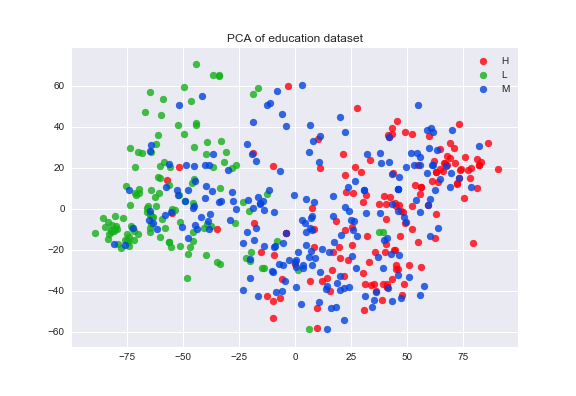## Analysis of Feature Selection Across Different Machine Learning Algorithms

For my machine learning class, my partner and I chose to do an analysis on how different machine learning algorithms handle feature selection and dimensionality reduction implicitly or explictly. We chose 4 machine learning models: support vector machines, Bayesian networks, and logistic regression with L1 and L2 norms. We compared the results to existing preprocessing techniques that are used for dimensionality reduction - Principle Component Analysis and Linear Discriminant Analysis. The linear transformation using PCA is shown below.Abstract

Feature importance and dimensionality reduction are important for effectively visualizing and interpreting real-world datasets, as well as improving prediction accuracy. Using the Students Academic Performance Dataset from Kaggle, we implemented support vector machines, Bayesian networks, and logistic regression with L1 and L2 norms. These algorithms with various hyperparamets were implemented to determine feature importance and predictive power of the models. These results were then compared to the important features determined from the preprocessing techniques: Principle Component Anaylsis (PCA) and Linear Discriminant Analysis (LDA). Using R2 values, which explain the percentage of the response variable explained by the variation in the model, it was found that L1 regularized logistic regression and Bayesian networks best fit the data. The higher R2 values identify that these algorithms had the most predictive power, allowing them to identify the ost important features in the dataset. SVM, LDA and L2 regularized logistic regression least accurately fit the data with SVM having the next highest R2 value and L1 having the lowerst. The important features between Bayesian networks, PCA, and LDA were compared, while the irrelevant features determined by SVM and L1/L2 logistic regression were also evaluated.

Moving forward, I would like to do more analysis in the sci-kit learn library feature_selection. I would also like to use multiple datasets and have more baselines to compare results to.